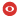Statut Confirmé Série IPN-THEO Domaines nucl-th Date Mercredi 29 Mai 2019 Heure 11:30 Institut IPN Salle Bâtiment 100, Salle A015 Nom de l'orateur Phillips Prenom de l'orateur D. R. Addresse email de l'orateur Institution de l'orateur Ohio University \newline Technische Universität Darmstadt Titre Insights into the reactions and structure of light nuclei from Effective Field Theory. Résumé In this talk I will show how an Effective Field Theory for weakly-bound few-body systems ("Halo EFT") aids the modeling and understanding of light nuclei in the $p$-shell. After introducing Halo EFT I will discuss two examples of its use. First, I will describe our recent analysis of the reaction $^3$He($\alpha$, $\gamma$)~. In this case we take $^3$He and $^4$He as the effective degrees of freedom and work to next-to-leading order in the EFT. We use a Bayesian analysis to perform the extrapolation of higher-energy data to solar energies and find a markedly smaller uncertainty to previous evaluations, as well as significant constraints on $^3$He-$^4$He scattering parameters. Second, I will demonstrate that a three-body ($\alpha$-neutron-proton) model of $^6$Li exhibits a correlation between the deuteron-$\alpha$ scattering length and the $^6$Li binding energy~. Such correlations appear generically in three-body systems with weak binding (cf. the Phillips line in the $A=3$ system) and can be understood as a consequence of the requirement that the EFT be renormalized. The emergence of such a correlation in the presence of $p$-wave nucleon-$\alpha$ interactions is particularly striking, as is the fact that it is rather insensitive to the actual values of the $p$-wave phase shifts. I will close by discussing the implications of this finding for model and EFT treatments of $^6$He and $^6$Li. Numéro de preprint arXiv Commentaires  Fichiers attachés 2019_05_29_D_Phillips.pdf (228396 bytes)Pour obtenir l' affiche de ce séminaire : [ Postscript | PDF ]

 [ Annonces ]    [ Abonnements ]    [ Archive ]    [ Aide ]    [ JavaScript requis ] [ English version ]